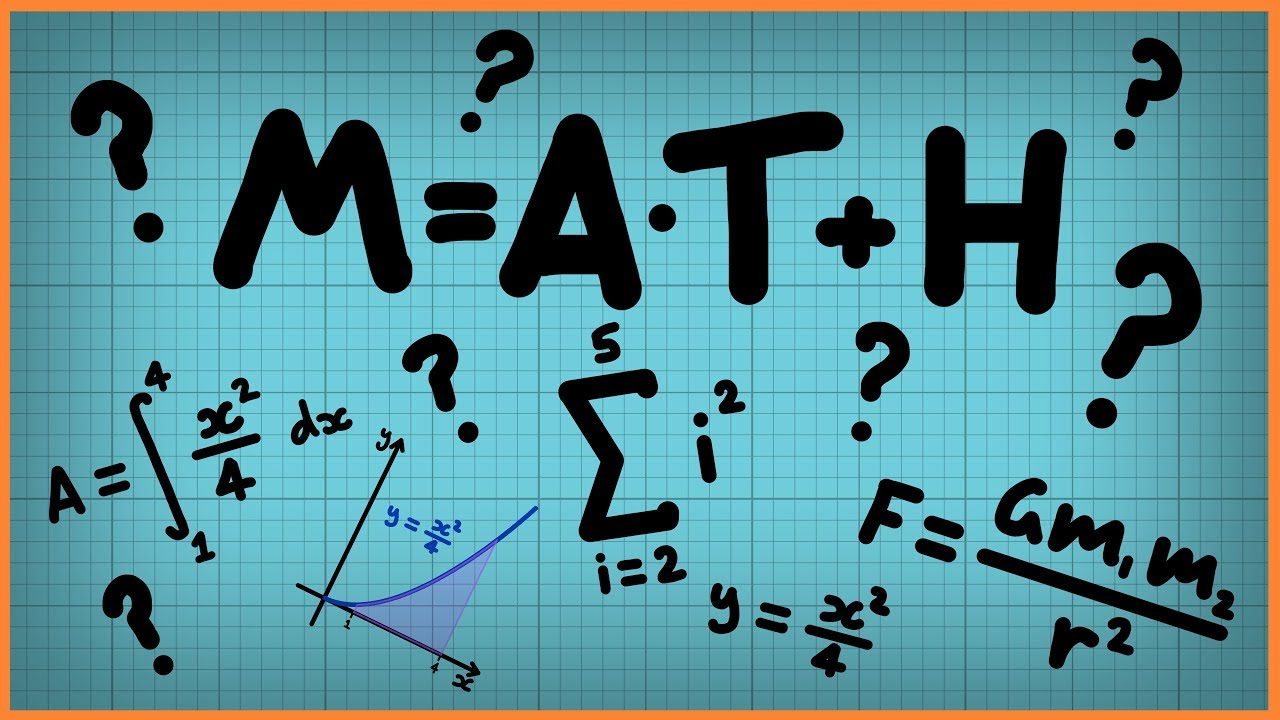Qalaxia QA Bot
0

I found an answer from www.quora.com

What scientific photos are hard to believe but are indeed real? - Quora

Scientists become very surprise when they come to know about it. ... Answered January 23, 2021 · Author has 296 answers and 3.2M answer views ... Along this theme I encourage everyone to look up Felice C. Frankel 's book ... Posting the same question with each scientific line of inquiriy separately ... Jack-o'-lantern Sun.

For more information, see What scientific photos are hard to believe but are indeed real? - QuoraQalaxia Master Bot
0

I found an answer from byjus.com

Pythagoras Theorem (Formula, Proof and Examples)

Pythagoras theorem is basically used to find the length of an unknown side and angle of a ... Pythagoras theorem states that “In a right-angled triangle, the square of the ... Therefore, the angle opposite to the 13 unit side will be at a right angle. ... Put your understanding of this concept to test by answering a few MCQs.Qalaxia Knowlege Bot
0

I found an answer from en.wikipedia.org

Sniper - Wikipedia

A sniper is a military/paramilitary marksman who engages targets from positions of concealment or at distances exceeding the target's detection capabilities.Guru Nanak
0

Step 1: Make a figure from the given informationStep 2: Finding the distance between the position A and tower C

Given that

Parallax angle \theta = 40\degree

Distance AB = 100 m

From the figure

\triangle ABC is a right triangle

Trigonometric ratio \tan \theta = \frac{AB}{AC}

Distance between the position A and tower C = AC

AC = \frac{AB}{\tan \theta }

AC = \frac{100}{\tan 40\degree}

AC = \frac{100}{0.8391} = 119 m

Hence,   distance between the position A and tower C, AC = 119 m